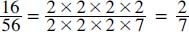NextPrevious

# How do you reduce a fraction?

To reduce a fraction, there are three general steps: factor the numerator, factor the denominator, and cancel out the fractional mixes that have the value of one. The leftover number is the reduced fraction. For example, to reduce 16/56, factor the numerator (16 = 2 × 2 × 2 × 2) and factor the denominator (56 = 2 × 2 × 2 × 7); eliminate the 2s (2/2 equals 1):Close

This is a web preview of the "The Handy Math Answer Book" app. Many features only work on your mobile device. If you like what you see, we hope you will consider buying. Get the App